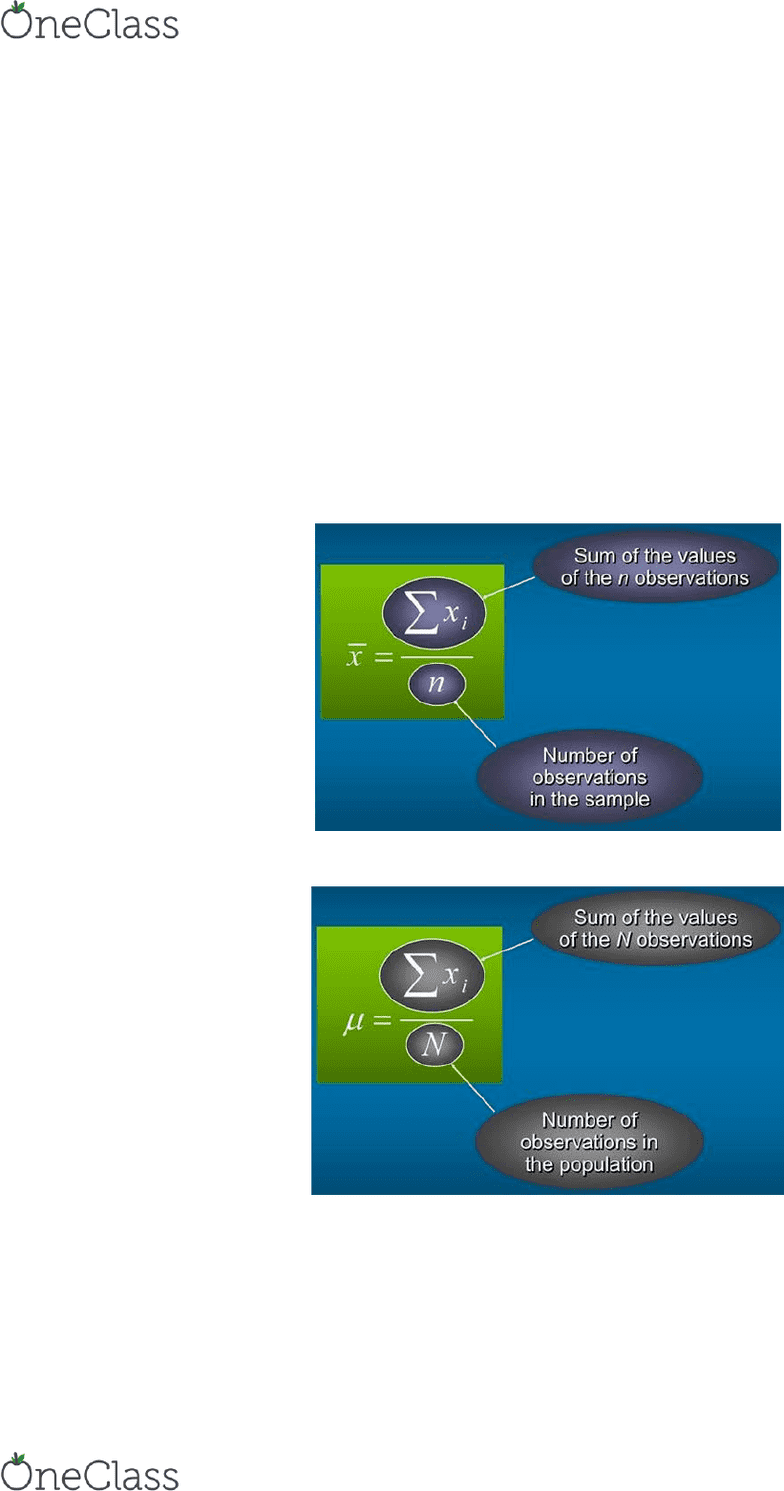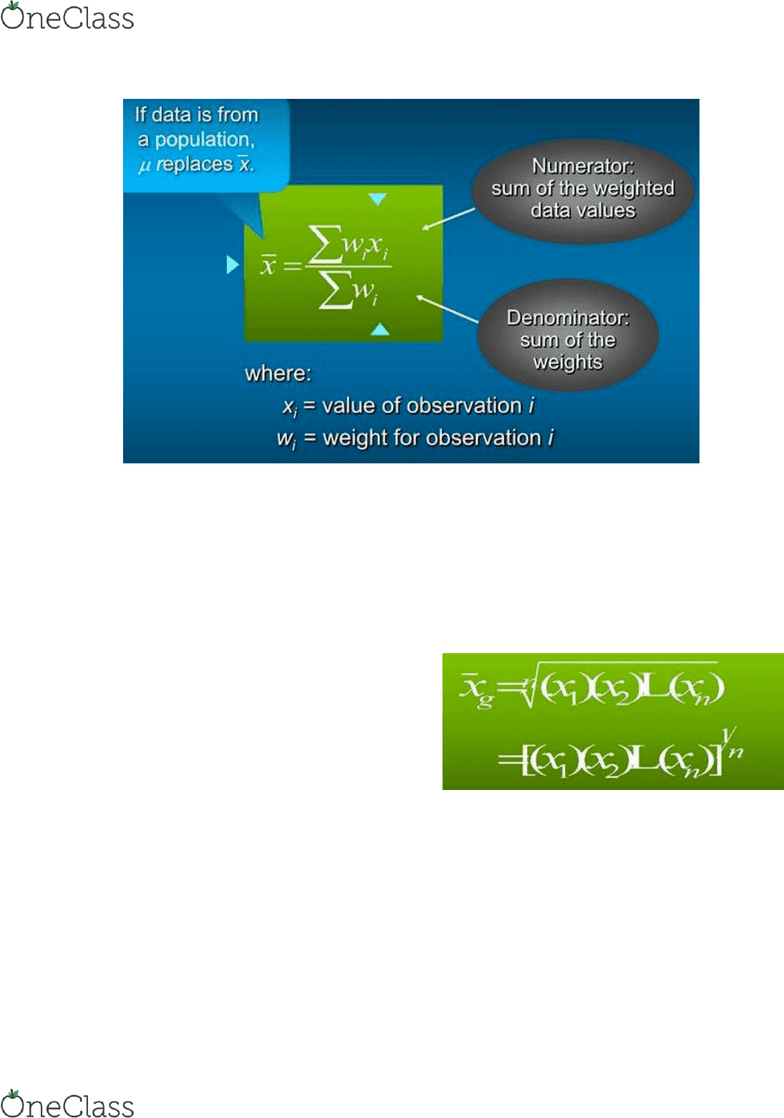Get 2 days of unlimited access
Class Notes (1,000,000)
CA (600,000)
UofGH (700)
(40)
Lecture 3

# SCMA*1000 Lecture Notes - Lecture 3: Summary Statistics, Percentile, Box Plot

Department
Course Code
SCMA*1000
Professor
Henk Brand
Lecture
3

This preview shows pages 1-2. to view the full 6 pages of the document.Week 3 - Chapter 3: Business Statistics Kevin Lu
Measures of Location:
- Mean
- Weighted Mean
- Median
- Geometric Mean
- Mode
- Percentiles
- Quartiles
Mean:
- The mean provides a measure of central location.
o The mean of data set is the average of all the data values
Sample Mean:
Population Mean:
Weighted Mean:
- The weights might be the number of credit hours earned for each grade, as in GPA.
find more resources at oneclass.com
find more resources at oneclass.com

Only pages 1-2 are available for preview. Some parts have been intentionally blurred.Week 3 - Chapter 3: Business Statistics Kevin Lu
Median:
- The median of a data set is the value in the middle when the data items are arranged in
ascending order.
Trimmed Mean:
- It is obtained by deleting a percentage of the smallest and largest values form a data set
and then computing the mean of the remaining values.
Geometric Mean:
- The geometric mean is calculated by
finding the nth root of the product of n
values.
Mode:
- The mode of a data et is the value that occurs with the greatest frequency
Percentiles:
- A percentile provides information about how the data are spread over the interval from
the smallest value to the largest value.
Quartiles
- Quartiles are specific percentiles
- First quartile = 25th Percentile
find more resources at oneclass.com
find more resources at oneclass.com
###### You're Reading a Preview

Unlock to view full version